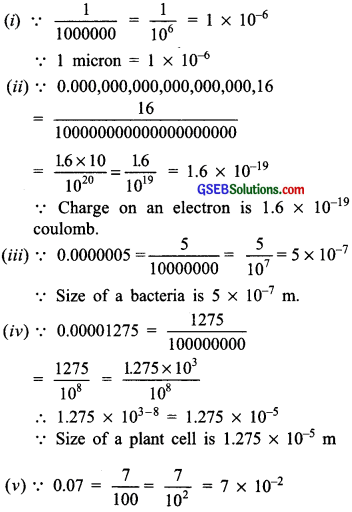# GSEB Solutions Class 8 Maths Chapter 12 Exponents and Powers Ex 12.2

Gujarat Board GSEB Textbook Solutions Class 8 Maths Chapter 12 Exponents and Powers Ex 12.2 Textbook Questions and Answers.

## Gujarat Board Textbook Solutions Class 8 Maths Chapter 12 Exponents and Powers Ex 12.2

Question 1.
Express the following numbers in standard form?

1. 0.0000000000085
2. 0.00000000000942
3. 6020000000000000
4. 0.00000000837
5. 31860000000

Solution:
1. 0.0000000000085 = $$\frac{85}{10000000000000}$$
= $$\frac{85}{10^{13}}$$ = $$\frac{8.5 \times 10}{10^{13}}$$ = 8.5 × 10 × 10-13
= 8.5 × 10-12

2. 0.00000000000942
= $$\frac{942}{100000000000000}=\frac{942}{10^{14}}=\frac{9.42 \times 10^{2}}{10^{14}}$$
= 9.42 × 102 × 10-14 = 9.42 × 10-12

3. 6020000000000000
= 602 × 10000000000000 = 602 × 1013
= 6.02 × 102 × 1013 = 6.02 × 1015

4. 0.00000000837 = $$\frac{837}{100000000000}=\frac{837}{10^{11}}$$
= $$\frac{8.37 \times 10^{2}}{10^{11}}$$ = 8.37 × 102 × 10-11
= 8.37 × 102+(-11) = 8.37 × 10-9

5. 3186000000 = 3186 × 1000000
= 3186 × 107 = 3.186 × 103 × 107
= 3186 × 103+7 = 3.186 × 1010Question 2.
Express the following numbers in usual form?
Solution:

1. 3.02 × 10-6
2. 4.5 × 104
3. 3 × 10-8
4. 1.0001 × 109
5. 5.8 × 1012
6. 3.61492 × 106

Solution:
1. 3.02 × 10-6 = 302 × 10-2 × 10-6
= 302 × 10-8= 361492 × 10 = 3614920

Question 3.
Express the number appearing in the following statements in standard form?

1. 1 micron is equal to $$\frac{1}{1000000}$$ m.
2. Charge of an electron is 0.000,000,000.000,000,000,16 coulomb.
3. Size of a bacteria is 0.0000005 m.
4. Size of a plant cell is 0.00001275 m.
5. Thickness of a thick paper is 0.07 mm.

Solution:∴ Thickness of a thick paper is 7 × 10-2 mm.Question 4.
In a stack there are 5 books each of thickness 20 mm and 5 paper sheets each of thickness 0.016 mm. What is the total thickness of the stack?
Solution:
∵ Thickness of one book = 20 mm
∴ Thickness of 5 books = 5 × 20 mm = 100 mm
Again,
Thickness of I paper sheet = 0.016 mm
∴ Thickness of 5 paper sheets
= 5 × 0,016 mm
= 0.080 m
∴ Total thickness = 100 mm + 0.080 mm
= 100.08 mm
= 1.0008 × 102 mm.